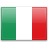S Q L T

S Q L T

S L T Q

S L Q T

S Q L T

S L T Q

S L Q T

S L Q T

S L Q T

S L Q T

S L T Q

S L Q T

S L T Q

Q T S L

S L Q T

S L Q T

L Q T S

S L Q T

L Q T S

L Q T S

L Q T S

L S T Q

S L T Q

L S T Q

L T S Q

Q S T

L T S

S T Q